Attention Model## RNN的局限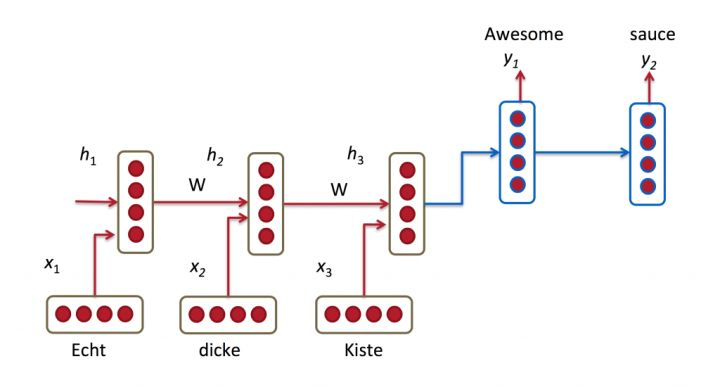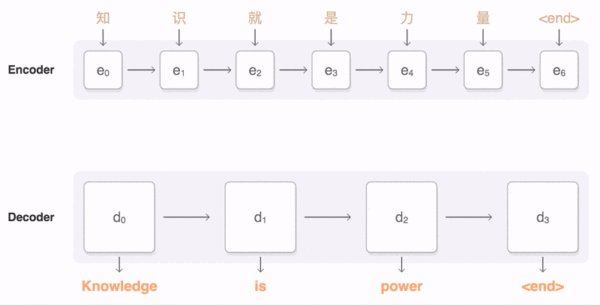## Encoder-Decoder框架Encoder-Decoder框架可以这么直观地去理解：可以把它看作适合处理由一个句子（或篇章）生成另外一个句子（或篇章）的通用处理模型。对于句子对 $< X, Y >$（例如 $X$ 是一个问句， $Y$ 是答案； $X$ 是一个句子， $Y$ 是抽取的关系三元组； $X$ 是汉语句子， $Y$ 是汉语句子的英文翻译等等），我们的目标是给定输入句子 $X$ ，期待通过Encoder-Decoder框架来生成目标句子 $Y$ 。 $X$ 和 $Y$ 分别由各自的单词序列构成：

## Attention Model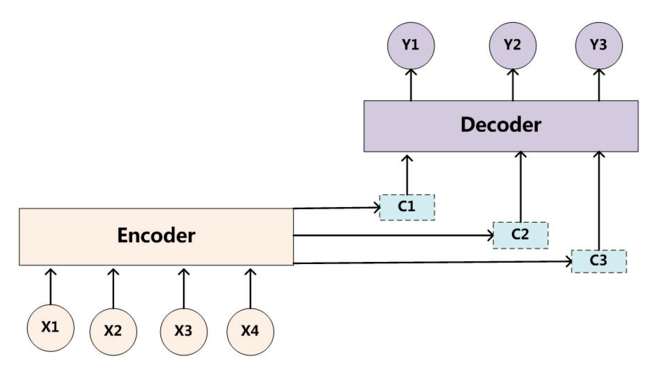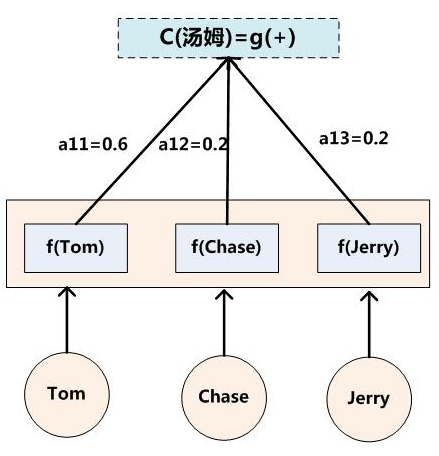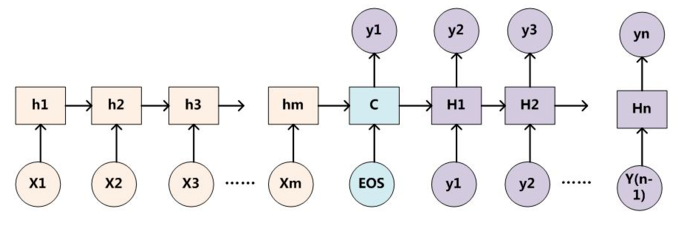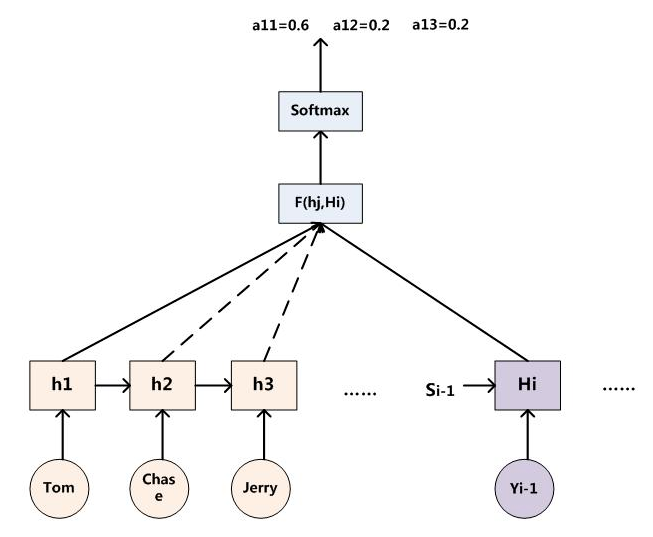### 数学表达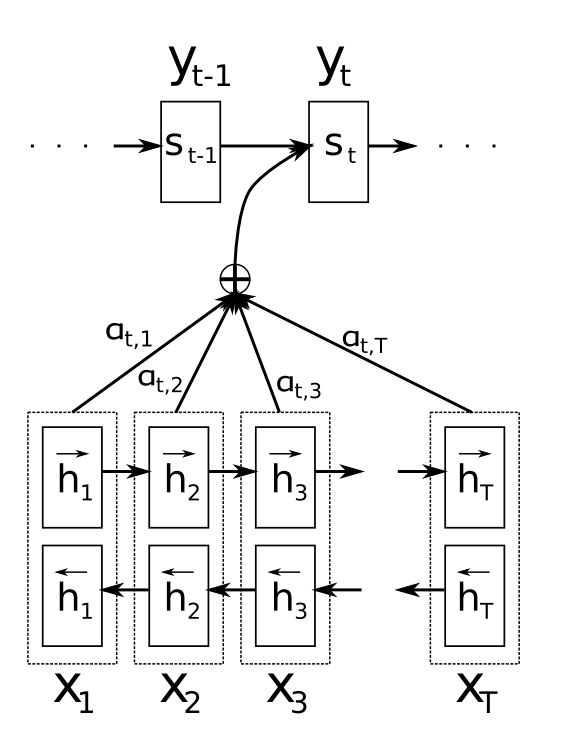1. 我们首先利用RNN结构得到encoder的hidden state $(h_1, h_2, \dots, h_T)$
2. 假设当前decoder的hidden state 是 $s_{t-1}$，我们可以计算每一个输入位置 $j$ 与当前输出位置的关联性，$e_{tj} = a(s_{t-1}, h_j)$ ，写成相应的向量形式即为 $\vec {e_t} = (a(s_{t-1}, h_1), a(s_{t-1}, h_2), \dots. a(s_{t-1}, h_T))$
3. 对 $\vec {e_t}$ 进行softmax操作得到attention的分布。$\vec {\alpha_t} = softmax(\vec {e_t})$，展开形式为 $\alpha_{tj} = \frac {e^{e_{tj}}} {\sum_{k=1}^T e^{e_{tk}}}$
4. 利用 $\vec {\alpha_t}$ 进行加权求和得到相应的context vector $\vec {c_t} = \sum_{j=1}^T \alpha_{tj}hj$
5. 由此，我们可以计算decoder的下一个hidden state $s_t = f(s_{t-1}, y_{t-1}, c_t)$ 以及该位置的输出 $p(y_t | y_1, \dots, y_{t-1}, \vec x) = g(y_{i-1}, s_t, c_t)$

### 本质思想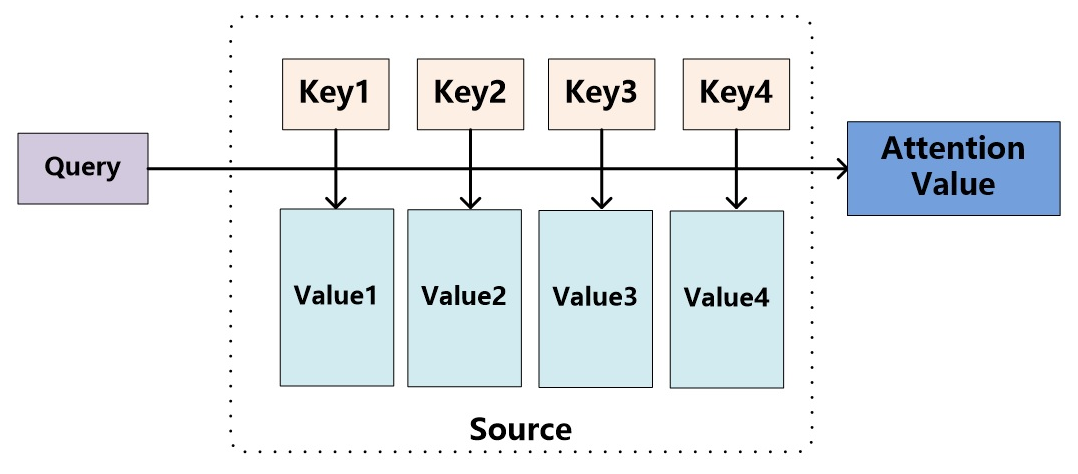$Lx$ 表示 Source 的长度，如一句话中单词的个数。上文所举的机器翻译的例子里，因为在计算Attention的过程中，Source中的Key和Value合二为一，指向的是同一个东西($h_i = f2$)，也即输入句子中每个单词对应的语义编码，所以可能不容易看出这种能够体现本质思想的结构。

AM的具体计算过程如下：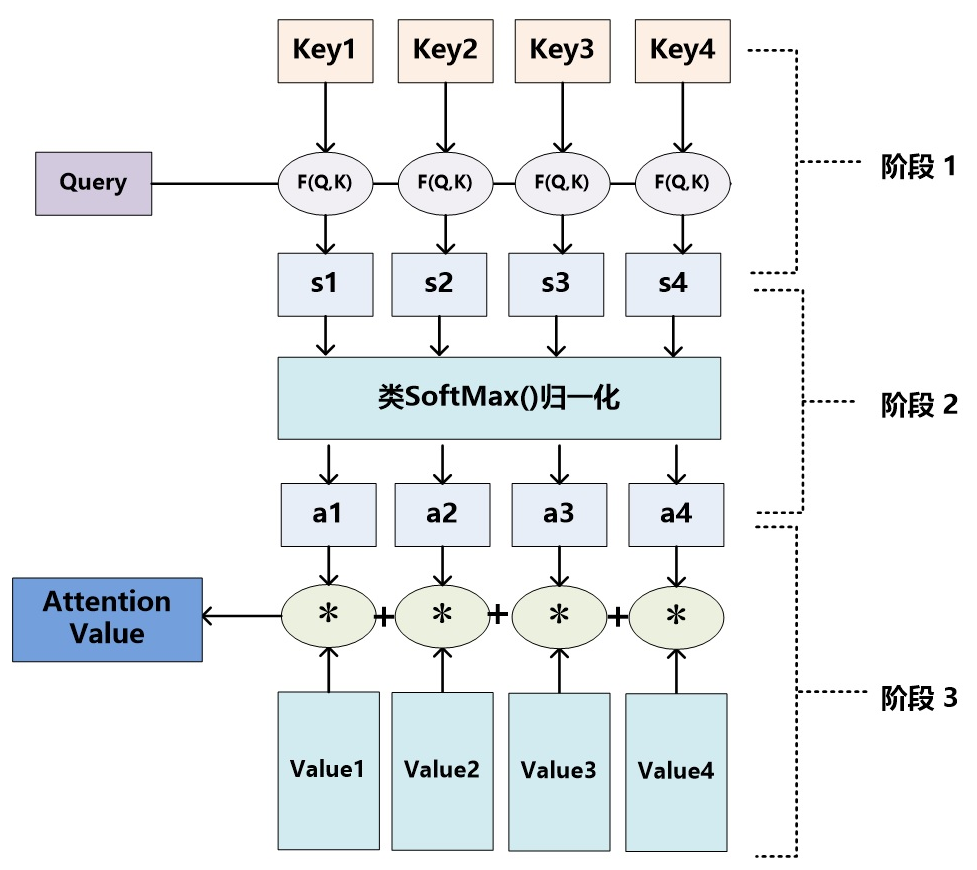Query和Key的相似性计算有如下常用几种方法：

• 点积：$Query \ast Key_i$
• cosine：$\frac { Query \ast key_i } { | Query | \ast | Key_i | }$
• 多层感知器：$MLP(Query, Key_i)$
• 欧式距离：$\sum_{j=1}^n (Query_j - Key_{ij})^2$